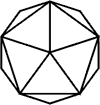## Regular Polyhedra

A regular polygon is a polygon with both equal side lengths and vertex angles.

Regular polyhedra are polygons with both identical regular faces and equal face-to-face angles.

There are five regular polyhedra: tetrahedron, cube, octahedron, icosahedron, dodecahedron.

 polyhedra vertices edges faces tetrahedron 4 6 4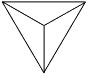cube 8 12 6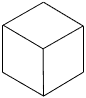octahedron 6 12 8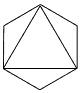dodecahedron 20 30 12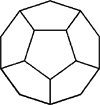icosahedron 12 30 20